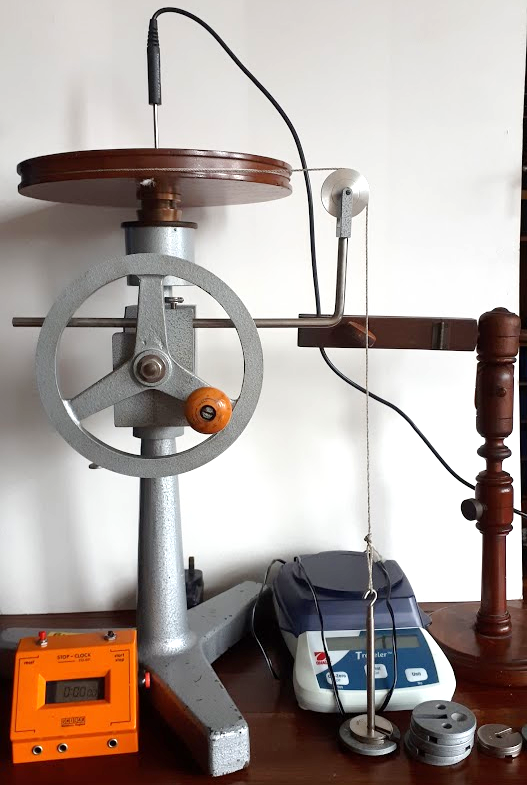# Heat From Friction

When two bodies pass over each other an force opposing the motion of the two bodies is produced called friction. As energy cannot be created or desrtoyed the energy taken from the motion of the objects is converted in heat. In this experiment a we investigate the heat produced by two bodies passing each other.

 DiagramTwo truncated cones of brass are machined so that one (C1) fits closely inside the other (C2), as shown.  A temperature sensor is inserted into a narrow hole drilled in the inner cone; this is connected to a computer and the temperature of the two-cone system can be read from the computer screen. A circular drum of radius R is attached to the inner cone and a piece of string is wound around the drum and then passed over a smooth pulley P and a mass m is attached to the end of the string so that it hangs vertically, as shown above.  If the lower brass cone were held fixed, release of the mass m would cause the string to unwind and the drum, and hence the inner brass cone, to rotate.

Alternatively, as in this experiment, the lower cone can be rotated by turning a wheel while the number of rotations made by the cone is recorded on a counter.  The wheel can be rotated smoothly at such a rate as to keep the mass m fixed in space, that is the forces due to the friction between the cones, transferred via the string, exactly balance the force of gravity on the mass m.  In this way a large number of rotations (N, say) can be completed which is equivalent to the mass m falling through a very great distance.

If the cones are thermally insulated so that the energy lost due to cooling during the experiment is negligible, it can be assumed that all of this mechanical work done goes into increasing the internal energy content of the system. As such the specific heat capacity of the cones, which are brass, can be calculated.

A similar experiment can be tried here: Mechanical Equivalent of Heat Lab

## Department of Physics

Roinn na Fisice

Room 213 (Physics Office), 2nd floor, Kane Science Building, University College Cork, Ireland.,

Top